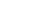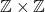How Cheenta works to ensure student success?
Explore the Back-Story

# Subgroups of(TIFR 2014 problem 16)Question:

How many subgroups does the group (\mathbb{Z\times Z}) have?

A. 1

B. 2

C. 3

D. infinitely many.

Discussion:

How many subgroups does (\mathbb{Z}) have? Well, for every (m\ge 0) we have a subgroup (m\mathbb{Z}).

And these are all distinct. So there are infinitely many subgroups of  (\mathbb{Z}).

So how many subgroups does (\mathbb{Z}\times {\bar{0}}) have? Again, for every (m\ge 0) we have a subgroup (m\mathbb{Z}\times {\bar{0}}). That is again infinitely many.

Since (\mathbb{Z}\times {\bar{0}}) is a subgroup of  (\mathbb{Z\times Z}) we have infinitely many subgroups of (\mathbb{Z\times Z}).

Question:

How many subgroups does the group (\mathbb{Z\times Z}) have?

A. 1

B. 2

C. 3

D. infinitely many.

Discussion:

How many subgroups does (\mathbb{Z}) have? Well, for every (m\ge 0) we have a subgroup (m\mathbb{Z}).

And these are all distinct. So there are infinitely many subgroups of  (\mathbb{Z}).

So how many subgroups does (\mathbb{Z}\times {\bar{0}}) have? Again, for every (m\ge 0) we have a subgroup (m\mathbb{Z}\times {\bar{0}}). That is again infinitely many.

Since (\mathbb{Z}\times {\bar{0}}) is a subgroup of  (\mathbb{Z\times Z}) we have infinitely many subgroups of (\mathbb{Z\times Z}).

This site uses Akismet to reduce spam. Learn how your comment data is processed.

### Knowledge Partner